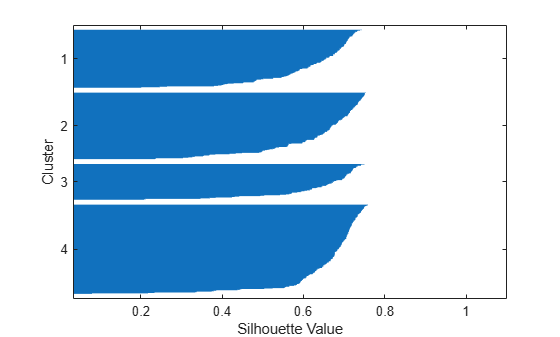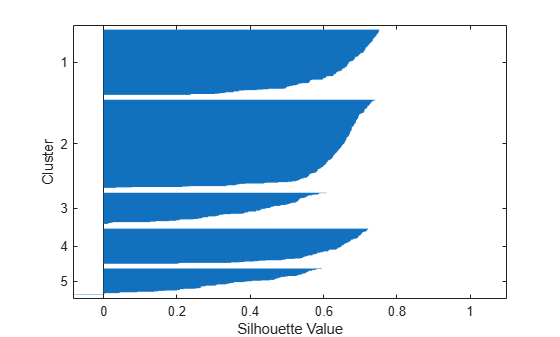## k-Means Clustering

This topic provides an introduction to k-means clustering and an example that uses the Statistics and Machine Learning Toolbox™ function `kmeans` to find the best clustering solution for a data set.

### Introduction to k-Means Clustering

k-means clustering is a partitioning method. The function `kmeans` partitions data into k mutually exclusive clusters and returns the index of the cluster to which it assigns each observation. `kmeans` treats each observation in your data as an object that has a location in space. The function finds a partition in which objects within each cluster are as close to each other as possible, and as far from objects in other clusters as possible. You can choose a distance metric to use with `kmeans` based on attributes of your data. Like many clustering methods, k-means clustering requires you to specify the number of clusters k before clustering.

Unlike hierarchical clustering, k-means clustering operates on actual observations rather than the dissimilarity between every pair of observations in the data. Also, k-means clustering creates a single level of clusters, rather than a multilevel hierarchy of clusters. Therefore, k-means clustering is often more suitable than hierarchical clustering for large amounts of data.

Each cluster in a k-means partition consists of member objects and a centroid (or center). In each cluster, `kmeans` minimizes the sum of the distances between the centroid and all member objects of the cluster. `kmeans` computes centroid clusters differently for the supported distance metrics. For details, see `'Distance'`.

You can control the details of the minimization using name-value pair arguments available to `kmeans`; for example, you can specify the initial values of the cluster centroids and the maximum number of iterations for the algorithm. By default, `kmeans` uses the k-means++ algorithm to initialize cluster centroids, and the squared Euclidean distance metric to determine distances.

When performing k-means clustering, follow these best practices:

• Compare k-means clustering solutions for different values of k to determine an optimal number of clusters for your data.

• Evaluate clustering solutions by examining silhouette plots and silhouette values. You can also use the `evalclusters` function to evaluate clustering solutions based on criteria such as gap values, silhouette values, Davies-Bouldin index values, and Calinski-Harabasz index values.

• Replicate clustering from different randomly selected centroids and return the solution with the lowest total sum of distances among all the replicates.

To perform k-means clustering interactively, use the Cluster Data Live Editor task.

### Compare k-Means Clustering Solutions

This example explores k-means clustering on a four-dimensional data set. The example shows how to determine the correct number of clusters for the data set by using silhouette plots and values to analyze the results of different k-means clustering solutions. The example also shows how to use the `'Replicates'` name-value pair argument to test a specified number of possible solutions and return the one with the lowest total sum of distances.

Load the `kmeansdata` data set.

```rng('default') % For reproducibility load('kmeansdata.mat') size(X)```
```ans = 1×2 560 4 ```

The data set is four-dimensional and cannot be visualized easily. However, `kmeans` enables you to investigate whether a group structure exists in the data.

Create Clusters and Examine Separation

Partition the data set into three clusters using k-means clustering. Specify the city block distance metric, and use the default k-means++ algorithm for cluster center initialization. Use the `'Display'` name-value pair argument to print the final sum of distances for the solution.

```[idx3,C,sumdist3] = kmeans(X,3,'Distance','cityblock', ... 'Display','final');```
```Replicate 1, 7 iterations, total sum of distances = 2459.98. Best total sum of distances = 2459.98 ```

`idx3` contains cluster indices that indicate the cluster assignment for each row in `X`. To see if the resulting clusters are well separated, you can create a silhouette plot.

A silhouette plot displays a measure of how close each point in one cluster is to points in the neighboring clusters. This measure ranges from 1 (indicating points that are very distant from neighboring clusters) through 0 (points that are not distinctly in one cluster or another) to –1 (points that are probably assigned to the wrong cluster). `silhouette` returns these values in its first output.

Create a silhouette plot from `idx3`. Specify `'cityblock'` for the distance metric to indicate that the k-means clustering is based on the sum of absolute differences.

```[silh3,h] = silhouette(X,idx3,'cityblock'); xlabel('Silhouette Value') ylabel('Cluster')```The silhouette plot shows that most points in the second cluster have a large silhouette value (greater than 0.6), indicating that the cluster is somewhat separated from neighboring clusters. However, the third cluster contains many points with low silhouette values, and the first and third clusters contain a few points with negative values, indicating that these two clusters are not well separated.

To see if `kmeans` can find a better grouping of the data, increase the number of clusters to four. Print information about each iteration by using the `'Display'` name-value pair argument.

`idx4 = kmeans(X,4,'Distance','cityblock','Display','iter');`
``` iter phase num sum 1 1 560 1792.72 2 1 6 1771.1 Best total sum of distances = 1771.1 ```

Create a silhouette plot for the four clusters.

```[silh4,h] = silhouette(X,idx4,'cityblock'); xlabel('Silhouette Value') ylabel('Cluster')```The silhouette plot indicates that these four clusters are better separated than the three clusters in the previous solution. You can take a more quantitative approach to comparing the two solutions by computing the average silhouette values for the two cases.

Compute the average silhouette values.

`cluster3 = mean(silh3)`
```cluster3 = 0.5352 ```
`cluster4 = mean(silh4)`
```cluster4 = 0.6400 ```

The average silhouette value of the four clusters is higher than the average value of the three clusters. These values support the conclusion represented in the silhouette plots.

Finally, find five clusters in the data. Create a silhouette plot and compute the average silhouette values for the five clusters.

`idx5 = kmeans(X,5,'Distance','cityblock','Display','final');`
```Replicate 1, 7 iterations, total sum of distances = 1647.26. Best total sum of distances = 1647.26 ```
```[silh5,h] = silhouette(X,idx5,'cityblock'); xlabel('Silhouette Value') ylabel('Cluster')````mean(silh5)`
```ans = 0.5721 ```

The silhouette plot indicates that five is probably not the right number of clusters, because two clusters contain points with mostly low silhouette values, and the fifth cluster contains a few points with negative values. Also, the average silhouette value for the five clusters is lower than the value for the four clusters. Without knowing how many clusters are in the data, it is a good idea to experiment with a range of values for `k`, the number of clusters.

Note that the sum of distances decreases as the number of clusters increases. For example, the sum of distances decreases from `2459.98` to `1771.1` to `1647.26` as the number of clusters increases from 3 to 4 to 5. Therefore, the sum of distances is not useful for determining the optimal number of clusters.

Avoid Local Minima

By default, `kmeans` begins the clustering process using a randomly selected set of initial centroid locations. The `kmeans` algorithm can converge to a solution that is a local (nonglobal) minimum; that is, `kmeans` can partition the data such that moving any single point to a different cluster increases the total sum of distances. However, as with many other types of numerical minimizations, the solution that `kmeans` reaches sometimes depends on the starting points. Therefore, other solutions (local minima) that have lower total sums of distances can exist for the data. You can use the `'Replicates'` name-value pair argument to test different solutions. When you specify more than one replicate, `kmeans` repeats the clustering process starting from different randomly selected centroids for each replicate, and returns the solution with the lowest total sum of distances among all the replicates.

Find four clusters in the data and replicate the clustering five times. Also, specify the city block distance metric, and use the `'Display'` name-value pair argument to print the final sum of distances for each solution.

```[idx4,cent4,sumdist] = kmeans(X,4,'Distance','cityblock', ... 'Display','final','Replicates',5); ```
```Replicate 1, 2 iterations, total sum of distances = 1771.1. Replicate 2, 3 iterations, total sum of distances = 1771.1. Replicate 3, 3 iterations, total sum of distances = 1771.1. Replicate 4, 6 iterations, total sum of distances = 2300.23. Replicate 5, 2 iterations, total sum of distances = 1771.1. Best total sum of distances = 1771.1 ```

In replicate 4, `kmeans` finds a local minimum. Because each replicate begins from a different randomly selected set of initial centroids, `kmeans` sometimes finds more than one local minimum. However, the final solution that `kmeans` returns is the one with the lowest total sum of distances over all replicates.

Find the total of the within-cluster sums of point-to-centroid distances for the final solution returned by `kmeans`.

`sum(sumdist)`
```ans = 1.7711e+03 ```